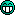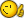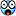# Need logic help with C/C++ question

#### SlashDK

##### MV VIP to the MAX
Hi people, I've come across this question which I am unable to solve. It is from INOI 2010. The sample input isn't very clear here, check it out at the original question paper.

Twin robots
You have a board divided into N×N squares and two robots, R2D2 and C3PO. Eachsquare on the board has a number written on it.
R2D2 starts at the top left corner square of the board and C3PO starts at the top right corner square of the board. Your aim is to move the robots so that R2D2 reaches the bottom right corner square and C3PO reaches the bottom left corner square. You have a remote control with two buttons, blue and yellow, that control the robots as follows.
• Whenever you press the blue button, R2D2 moves one square to the right and C3PO moves one square down.
• Whenever you press the yellow button, R2D2 moves one square down and C3PO moves one square left.
The robots are not allowed to move off the board—if pushing a button would force either one of the robots to move off the board, the button push has no effect and both robots stay where they are.
For each robot, you compute a score which is the sum of the numbers on the squares that it visits along the path that it follows from its starting position to its final position. The combined score of the two robots is the sum of their individual scores. Your aim is to compute the maximum combined score that the two robots can achieve.
For example, suppose you have a 4 × 4 board as follows.
6 0 3 −1
7 4 2 4
−3 3 −2 8
13 10 −1 −4
In this example, if R2D2 follows the path marked by ∗ and C3PO follows the path marked by †, as shown below, their combined score is 56.
6∗ 0 3† −1†
7∗ 4∗† 2† 4
−3 3∗† −2∗ 8∗
13† 10† −1 −4∗
It can be verified that this is the best combined score that can be achieved for this board.

I've managed to make functions for the movements and adding the achieved values, but I am not able to think of a logic that would go through all possible paths through the array.

#### vickybat

##### I am the night...I am...
^^ The question was very tricky and it took me a while to figure it out. I've written the code in java using the sample inputs given and got the desired maximum output. It can be generalized into any N x N array filled with elements by user inputs.
Just check it out mate to see if you're getting the logic i've used. If not, i'll explain in detail.
I've used java simply coz i'm comfortable with it. You can easily port it to c++ or any language.

For now i've combined the buttons in a single function. It can be modified as you wish it to work.Code:
``````[B]public class TwinRobots {
int R2D2 ;
int C3PO ;
int i = 0;
int j = 0;
int k = 0;
int l = 3;
int t1[][] = new int[][] {{6,0,3,-1},{7,4,2,4},{-3,3,-2,8},{13,10,-1,-4}};

public static void main (String[] args){
TwinRobots tw = new TwinRobots();
tw.check();
}
public TwinRobots () {

R2D2 = t1[i][j];
C3PO = t1[k][l];
}

public void check(){

int a = t1[i][j+1];
int b = t1[i+1][j];

int[] arr_len1 = new int ;
int[] arr_len2 = new int;

while( t1[i][j] != t1){

arr_len1[i] = t1[i];

arr_len2[j] = t1[j];

if (b>=a && (t1[i][j] - arr_len1[i] >= t1[i][j] - arr_len2[j]) ) {

R2D2 += t1[i+1][j];
C3PO += t1 [k][l-1];

if ( i < 2 && l > 0){
i++;
l--;

} else {
i = 3;
l = 0;
}

a = t1[i][j+1];
b = t1[i+1][j];

}

else  {
R2D2 += t1[i][j+1];
C3PO += t1[k+1][l];

if (j < 2  && k < 2){
j++;
k++;
} else {

j = 3;
k = 3;

R2D2 += t1[i+1][j];
C3PO += t1 [k][l-1];
}
if (j > 2 ){
a=0;
b=0;

}   else{
a = t1[i][j+1];
b = t1[i+1][j];

}

}

}
System.out.print("output is:" );
System.out.println (R2D2+C3PO);

}

}[/B]``````

#### SlashDK

##### MV VIP to the MAX
Thanks for the reply, although I figured out the logic a few days after posting this problem. I'm mainly a C++ programmer but looking at your logic, from what I understand, it may not work for larger values of n. Watch the path counting brain teaser at Khan Academy, it will show you where the logic goes wrong. I'll be posting the logic that I made soon. I used a partially greedy algorithm to find the maximum score for each position in the first row and column and then each row and column to below and to the right of the current ones.

#### vickybat

##### I am the night...I am...
Thanks for the reply, although I figured out the logic a few days after posting this problem. I'm mainly a C++ programmer but looking at your logic, from what I understand, it may not work for larger values of n. Watch the path counting brain teaser at Khan Academy, it will show you where the logic goes wrong. I'll be posting the logic that I made soon. I used a partially greedy algorithm to find the maximum score for each position in the first row and column and then each row and column to below and to the right of the current ones.

Do test the above code with large values. It should work. Just replace 3 and 2 with N & N-1.
Do post the findings as well as your logic mate. I want to take a look at it.

Btw i'm not from a computer science background but electronics and telecommunications. Algorithms were never my forte but i have developed a deep interest in it as well as programming in recent times. I could have posted that in c++ but when i was thinking about the problem from scratch, i could only think in java from constructors, object creation, multidimensional arrays and almost everything. All these things mentioned are implemented in the above code.

And thanks to you for introducing INOI problems in TDF. They are challenging and i wonder how those guys could solve two questions within 3 hrs. I'm way behind them in this aspect.Please do post your code mate.#### SlashDK

##### MV VIP to the MAX
Sorry for not posting my solution for so long. Couldn't find it since I forgot the computer on which I had saved it on. But here it is -
Code:
``````#include<iostream>
using namespace std;
int main()
{
int k,a,b,x=0,y=0,xt=0,yt=0;
cout<<"Enter n";
cin>>k;
const int n=k;
cout<<"Enter values";
int varr[n][n], sarr[n][n],sarrt[n][n],larr[n];
for(b=0;b<k;b++)
{
for(a=0;a<k;a++)
{
cin>>varr[b][a];
}
}
xt=k-1;
sarr=varr;
sarr[xt]=varr[xt];
sarrt[xt]=varr[xt];
larr=varr[xt];
for(a=1;a<k;a++)
{
larr[a]=varr[a][k-1]+larr[a-1];
}
for(x=xt-1;x>=0;x--)
{
sarrt[x]=varr[x]+sarrt[x+1];
}

for(y=1;y<k;y++)
{
sarr[y]=varr[y]+sarr[y-1];
}
for(y=1;y<k;y++)
{
sarr[y][xt]=varr[y][xt]+sarr[y-1][xt];
}
for(x=1;x<k;x++)
{
sarr[x]=varr[x]+sarr[x-1];
}
for(x=1;x<k;x++)
{
sarr[x]=sarr[x]+larr[x];
}
for(y=1;y<k;y++)
{
sarr[y]=sarr[y]+sarrt[xt-y];
}
xt=k-1;
yt=1;
for(y=1;y<k;y++)
{
for(x=1;x<k;x++)
{
if(sarr[y-1][x]>sarr[y][x-1])
{
sarr[y][x]=varr[y][x]+sarr[y-1][x]+varr[yt][xt];
}
else if(sarr[y-1][x]<sarr[y][x-1])
{
sarr[y][x]=varr[y][x]+sarr[y][x-1]+varr[yt][xt];
}
else
{
sarr[y][x]=varr[y][x]+sarr[y-1][x]+varr[yt][xt];
}
yt++;
}
if(xt!=0)
xt--;
}
cout<<sarr[k-1][k-1];
}``````

This should work for all possible values according to me. The logic is the same as I posted before.

Oh and I'm not YET from a computer science background since I'm still in class 10#### dashing.sujay

##### Moving
Staff member
^ You're going very good. I'm telling you, 80% of CS engg students are not of the level to solve the above program. Keep it up#### vickybat

##### I am the night...I am...
@ SlashDK

Are you serious ? You are in class 10 and solving INOI problems ??Boy you are good in programming no doubt. When i was in class 10, we had "basic" and i was very weak in it.
I think your interest fuels the passion for coding. Never let it dry down.You are indeed very good, good enough to teach me a thing or two.I will go through your logic mate. It differs from mine a lot but i'll check using different inputs.
Have you tested the above code with the sample input?

P.S - It would be great if you assign variable names as per question (like R2D2 AND C3PO for the robots in this case). This makes code readability a lot easier and also to debug.^ You're going very good. I'm telling you, 80% of CS engg students are not of the level to solve the above program. Keep it upConsidering the teaching standards and overall curriculum these days in b-tech, i guess you are absolutely right.Only interested students can do well. Programming is not something that can be force fed which happens in colleges these days.

#### SlashDK

##### MV VIP to the MAX
Thanks for the compliments. And sorry for not putting proper variable names in the code. All the x and y's refer to coordinates. I haven't done separate scores for both the robots since I though it would be easier if i just took both their scores together all the time since that's whats supposed to be done anyway. As I said before, the code calculates maximum score for each position in the first row and first column and then each row and column to below and to the right of the current ones, thereby giving the maximum score for the final position.

and BTW INOI is meant for class 8-12 students. Sadly my INOI went very poorly since it was the first time I gave it and I was totally out of my comfort zone even though I could easily make the complete logic for both the questions.